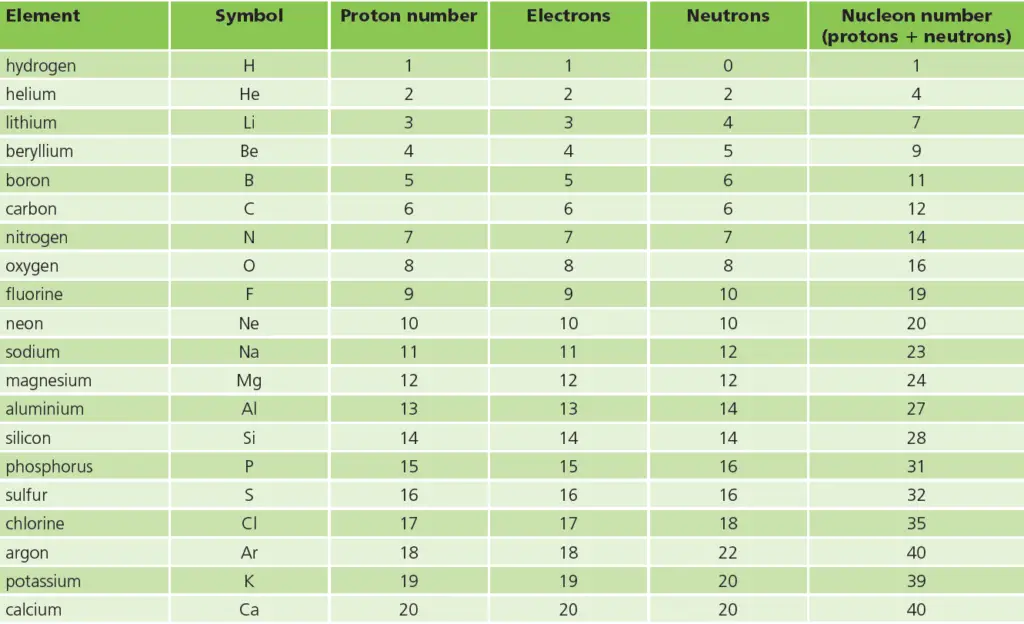# The atoms of the first 20 elements in the periodic table

Last updated on November 15th, 2021 at 03:15 pm

In the Periodic Table, the elements are arranged in order of increasing proton number. Here is a quick list of the names of the first 20 elements in the periodic table: hydrogen, helium, lithium, beryllium, boron, carbon, nitrogen, oxygen, fluorine, neon, sodium, magnesium, aluminium, silicon, phosphorus, sulphur, chlorine, argon, potassium, & calcium.

In this post, we have listed some important facts of these first 20 elements in tabular format. See the image below for the list. This list contains the element name, the symbol of the element, number of protons, number of electrons, number of neutrons, & nucleon number of each of these 20 elements.The atoms of the first 20 elements: The list contains the element name, the symbol of the element, number of protons, number of electrons, number of neutrons, & nucleon number of each of these 20 elements.

So the numbers of protons and electrons increase by 1 at a time – and are always equal.

## Atoms – FAQs

Atoms consist of a nucleus and a cloud of electrons that move around the nucleus. The nucleus is itself a cluster of two kinds of particles, protons, and neutrons.

All the particles in an atom are very light. So their mass is measured in atomic mass units, rather than grams. Protons and electrons also have an electric charge.

The number of protons in an atom is called its proton number. The proton number of sodium is 11.

Every atom has an equal number of protons and electrons. So atoms have no overall charge.

The total number of protons and neutrons in an atom is called its nucleon number.
The nucleon number for the sodium atom is 23. (11 +12 = 23)